# Sample Data: London Cholera

Locations of the 1854 London cholera outbreak near Golden Square annotated with marks including the number of cases, distance (in meters) to the contaminated Broad Street water pump, distance (in meters) to a non-Broad Street pump, and whether or not the Broad Street pump was the closest pump

## Details

Locations of the 1854 London cholera outbreak near Golden Square in the observation region GeoBoundsRegion[{{51.51065909836119`, 51.51581097178905`}, {-0.14008439736259973`, -0.13222907147321172`}}], annotated with marks including the number of cases, distance (in meters) to the contaminated Broad Street water pump, distance (in meters) to a non-Broad Street pump, and whether or not the Broad Street pump was the closest pump.

## Examples

### Basic Examples (2)

Retrieve the data:

 In:=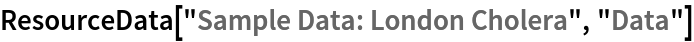Out=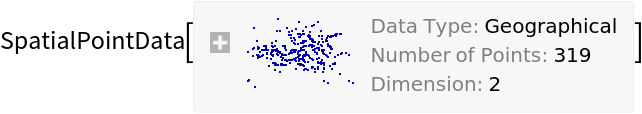Summary of the spatial point data:

 In:=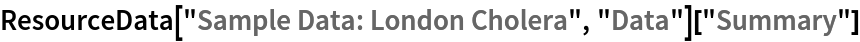Out=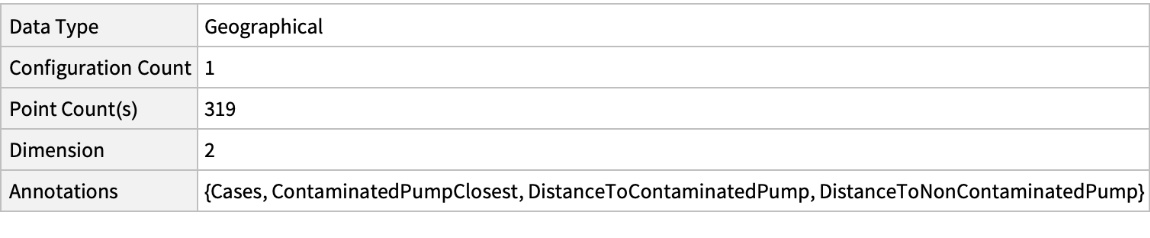### Visualizations (2)

Plot the locations:

 In:=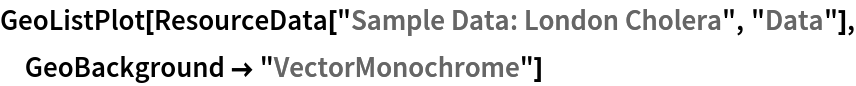Out=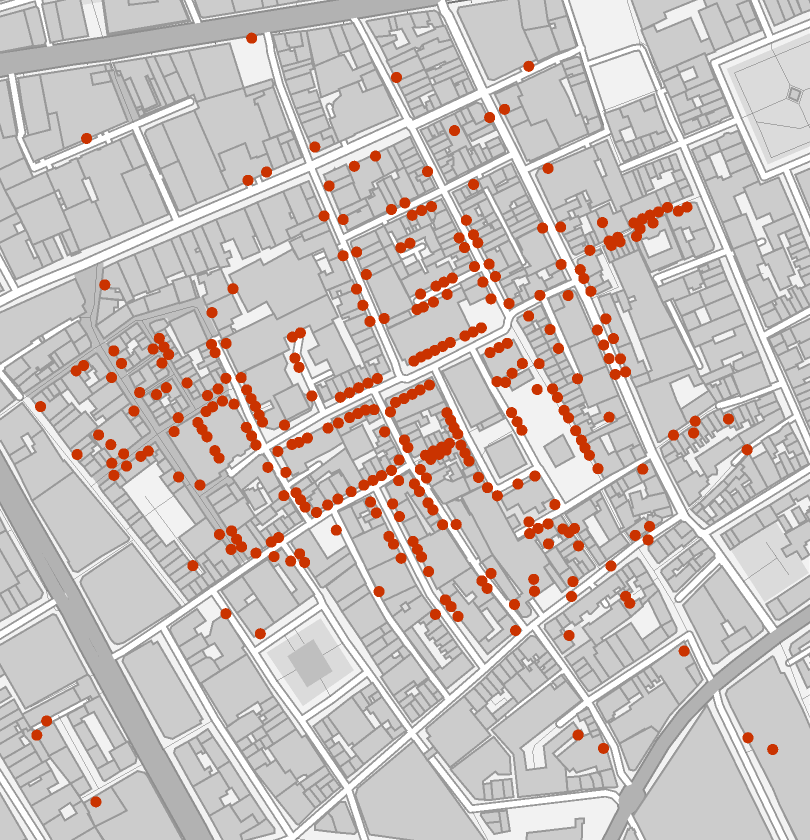Visualize the number of cases per location with information whether the contaminated pump was the closest:

 In:=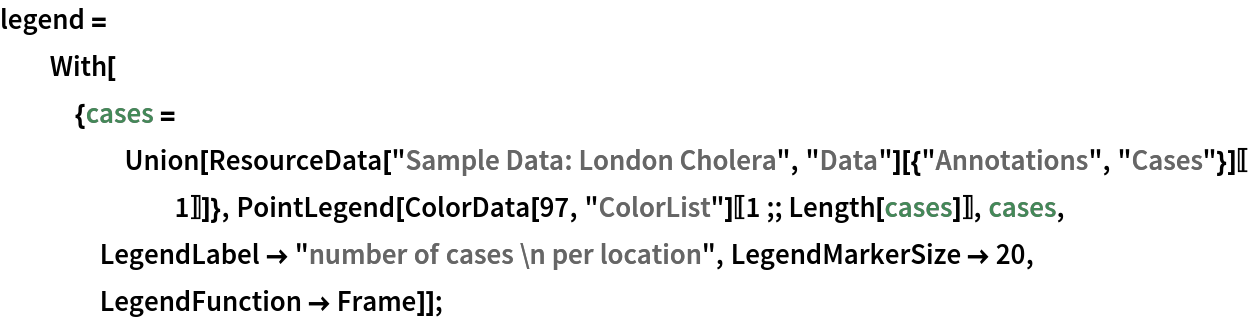In:=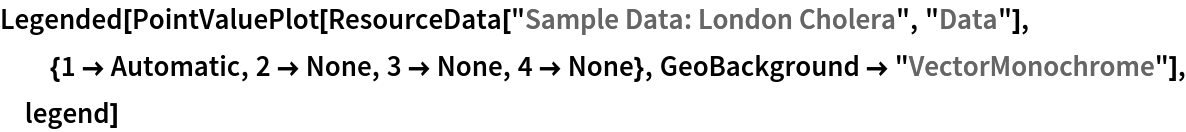Out=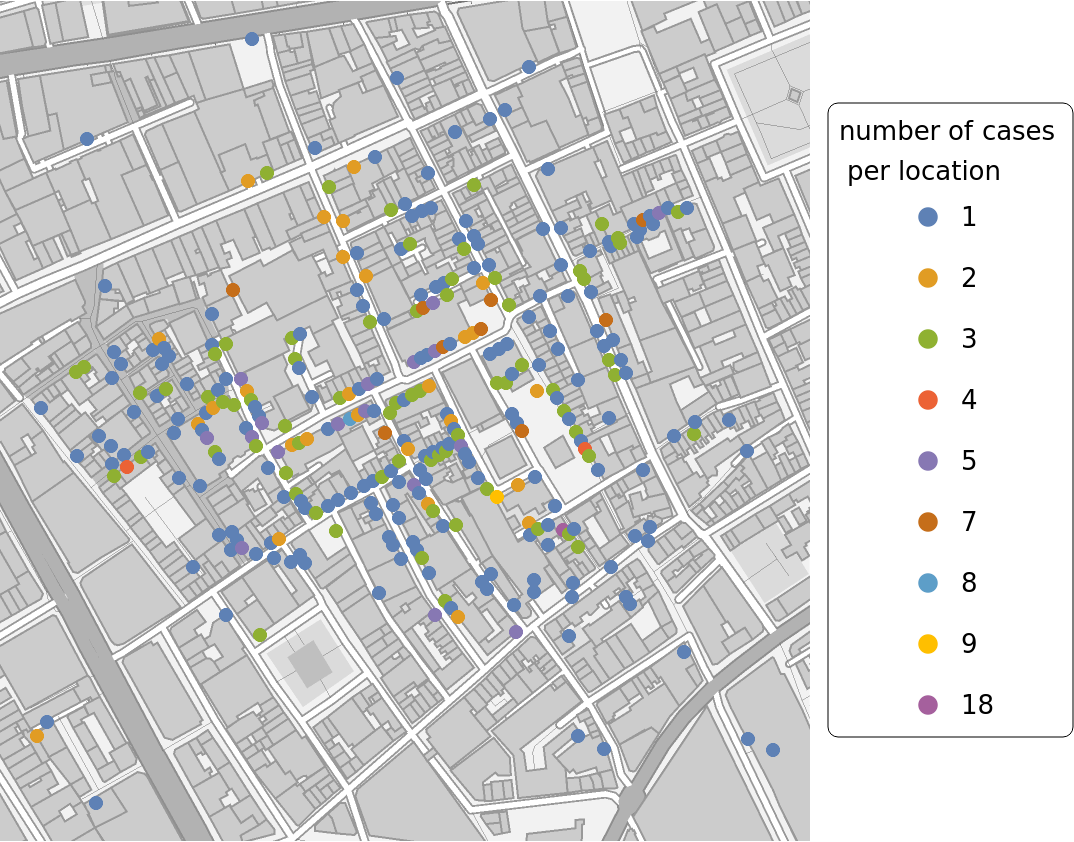### Analysis (3)

Compute probability of finding a point within given radius of an existing point - NearestNeighborG is the CDF of the nearest neighbor distribution:

 In:=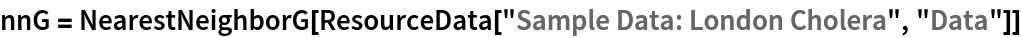Out=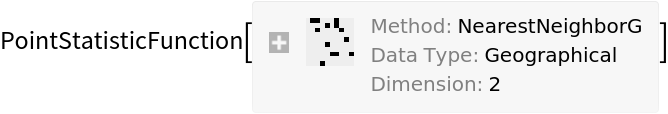In:=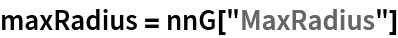Out=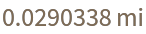In:=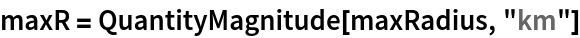Out=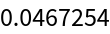In:=Out=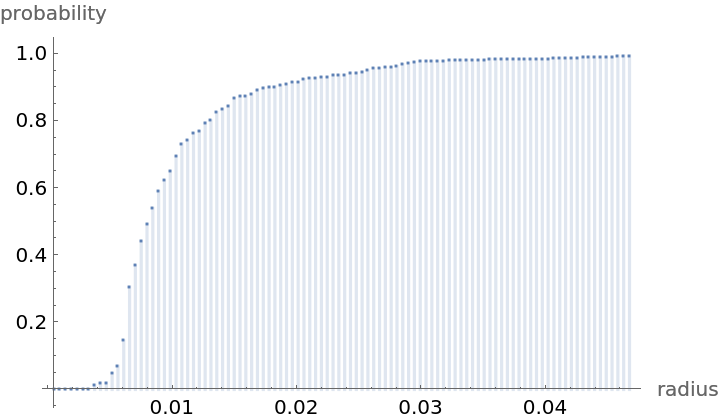Mean distance between a typical point and its nearest neighbor (for positive support distribution can be approximated via a Riemann sum of 1-CDF):

 In:=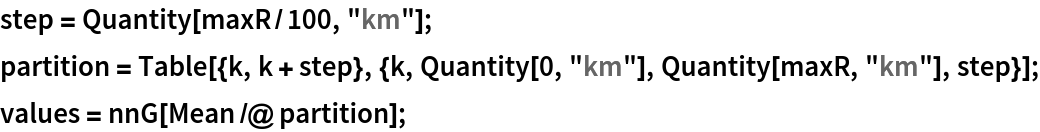In:=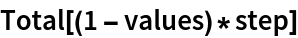Out=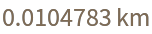Test for complete spacial randomness:

 In:=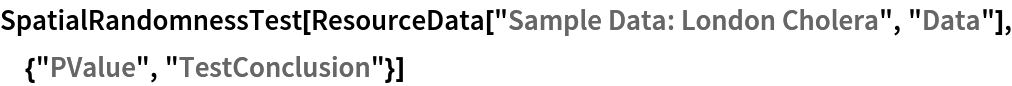Out=Gosia Konwerska, "Sample Data: London Cholera" from the Wolfram Data Repository (2021)